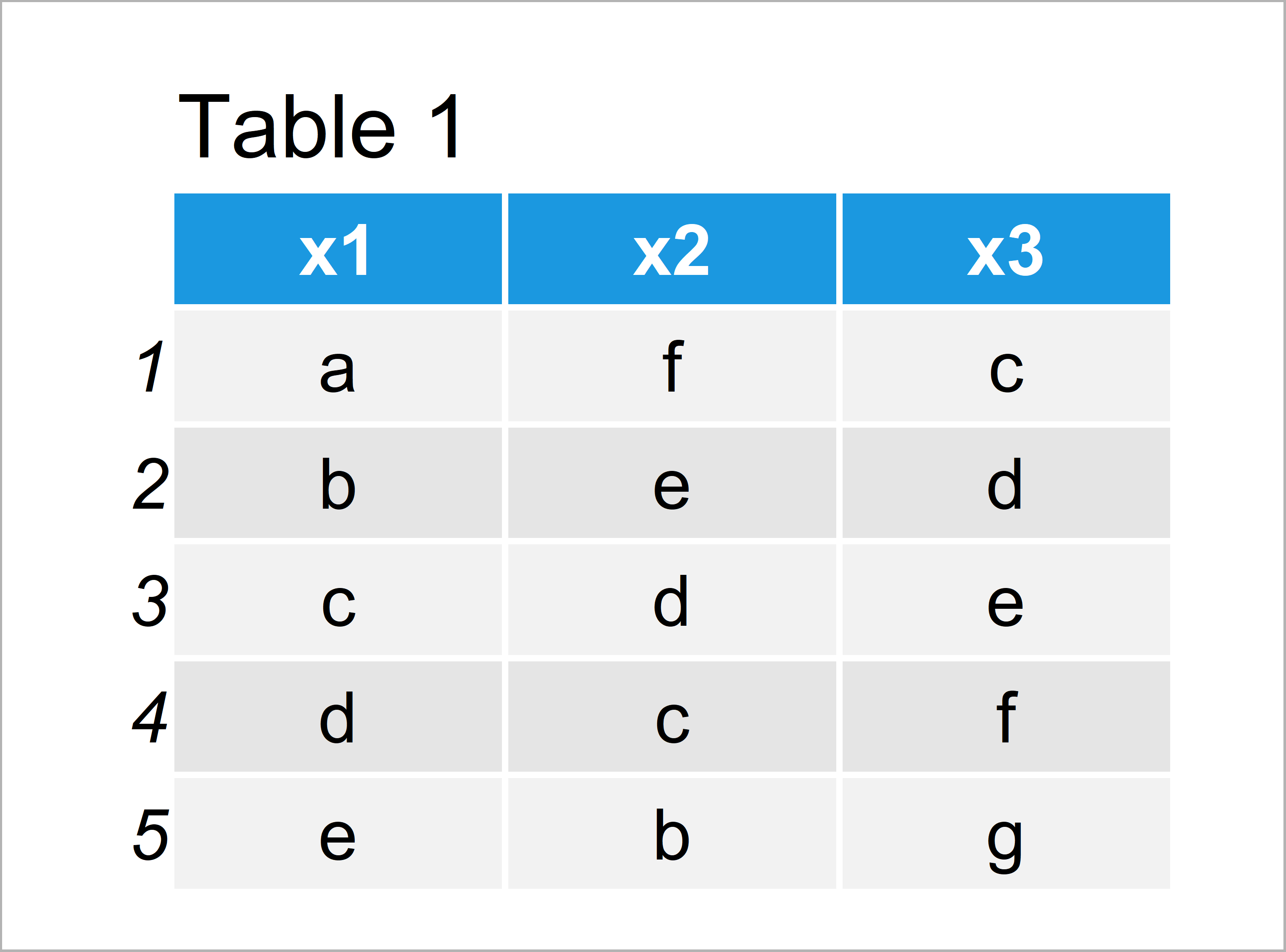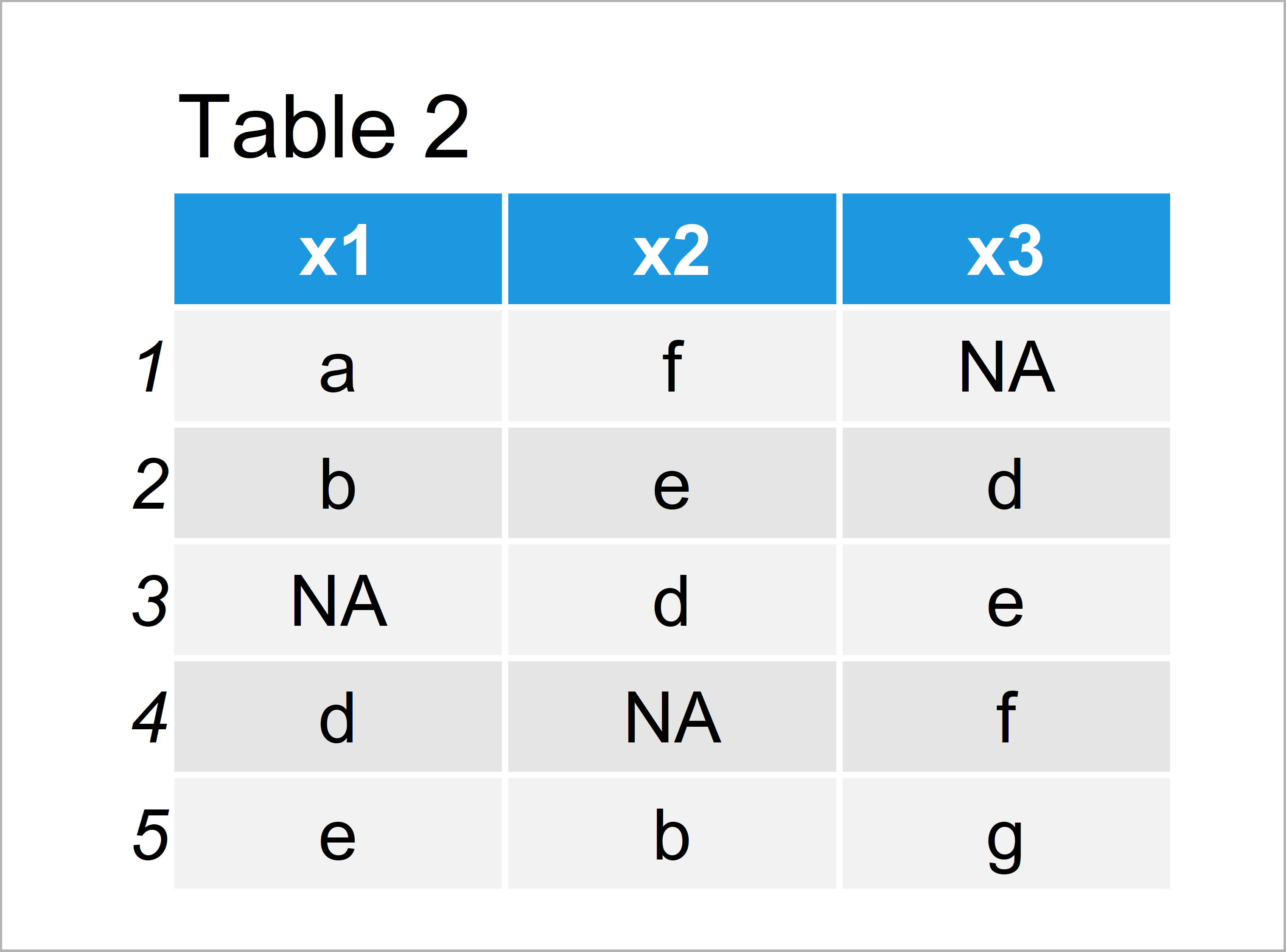# Replace Character Value with NA in R (2 Examples)

In this tutorial, I’ll show how to replace characters in vectors and data frame columns by NA in the R programming language.

Let’s get started!

## Example 1: Replace Specific Character Value with NA in Vector

In Example 1, I’ll illustrate how to set particular characters in a vector to NA (i.e. missing value).

Consider the following example vector in R:

```vec <- c("A", "B", "A", "C", "B") # Create example vector vec # Print example vector #  "A" "B" "A" "C" "B"```

As you can see based on the previous output of the RStudio console, our example vector contains a sequence of uppercase letters.

Let’s assume that we want to exchange the character letter “A” by NA. Then, we can use the following R code:

```vec_NA <- vec # Duplicate vector vec_NA[vec_NA == "A"] <- NA # Replace particular value with NA vec_NA # Print updated vector #  NA "B" NA "C" "B"```

Have a look at the previous output: We have created a new character vector called vec_NA that contains Not Available values at the index positions where our input vector had the character value “A”.

## Example 2: Replace Specific Character Value with NA in Data Frame

In this example, I’ll show how to replace characters in data frame variables by NA.

First, we have to create some example data:

```data <- data.frame(x1 = letters[1:5], # Create example data frame x2 = letters[6:2], x3 = letters[3:7]) data # Print example data frame```As shown in Table 1, the previous R syntax has created a data frame with five rows and three columns. All the columns contain characters.

Now, we can replace a particular character value (i.e. “c”) with NA using a logical condition:

```data_NA <- data # Duplicate data frame data_NA[data_NA == "c"] <- NA # Replace particular value with NA data_NA # Print updated data frame```In Table 2 it is shown that we have created a new data frame containing NA values using the previous R programming code.

## Video & Further Resources

Please accept YouTube cookies to play this video. By accepting you will be accessing content from YouTube, a service provided by an external third party.If you accept this notice, your choice will be saved and the page will refresh.

In addition, you may want to have a look at the other articles on my website. I have released several related tutorials on topics such as dplyr, merging, and missing data.